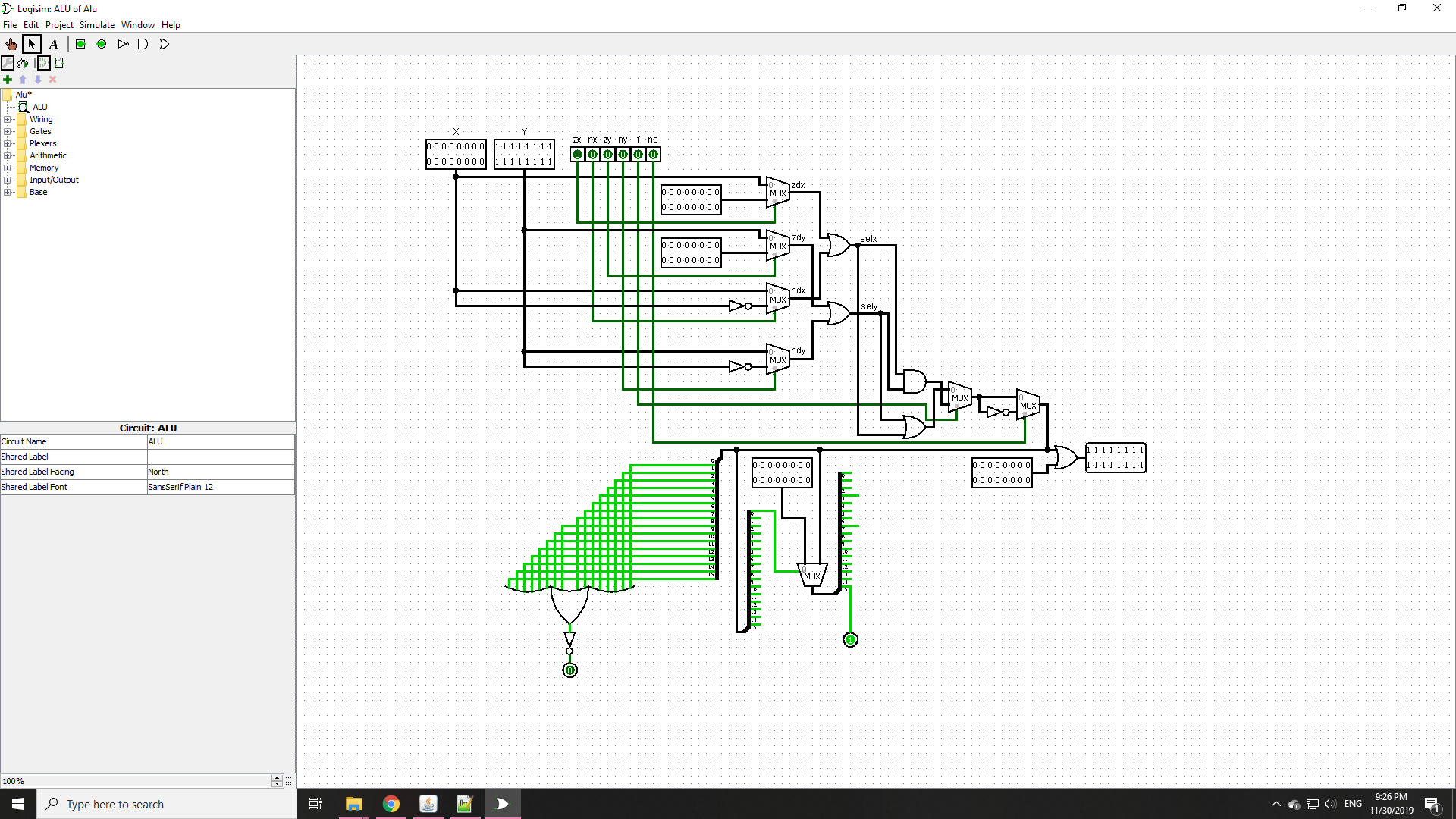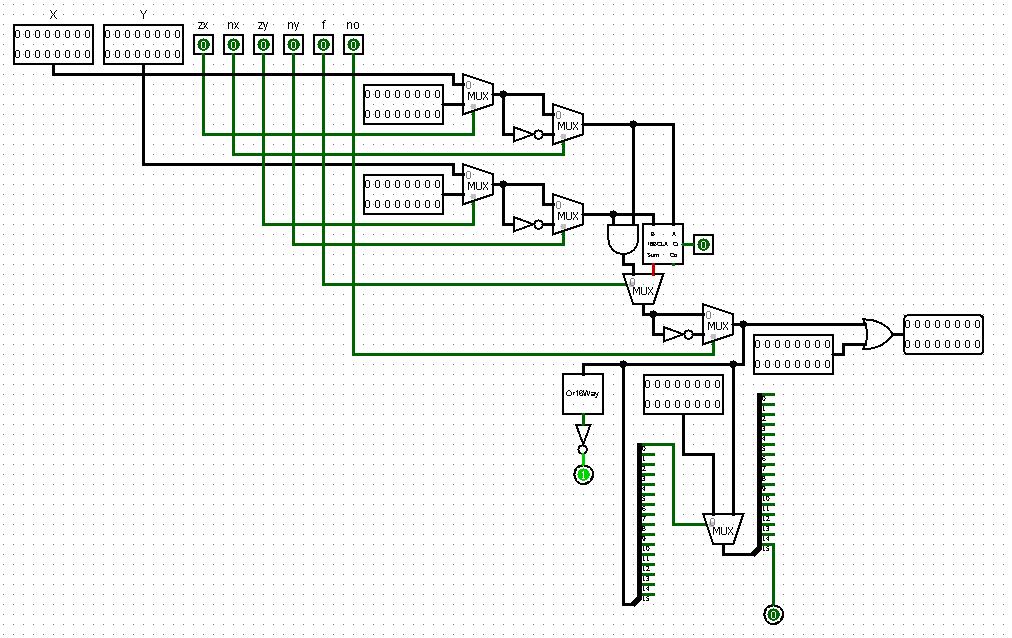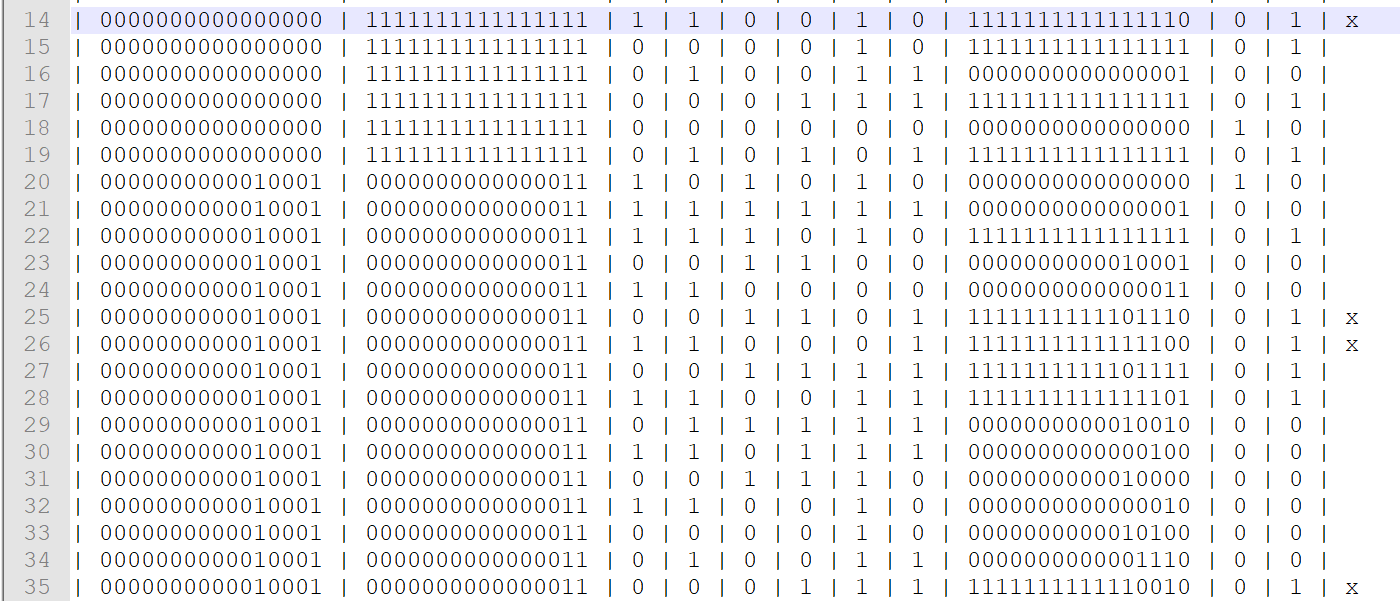ClassicListThreaded4 messagesOpen this post in threaded view
|

 This post was updated on . Hi guys I'm getting an error trying to load my ALU. The error I'm getting is: An internal pin may only be fed once by a parts output... My Parts are as follows:     PARTS:    // Put you code here:    //Zero the x input    Mux16(a = x, b[0..15] = false, sel = zx, out = zdx);        //Negate the x input    Not16(in = x, out = notx);    Mux16(a = x, b = notx, sel = nx, out = ndx);        //Zero the y input    Mux16(a = y, b[0..15] = false, sel = zy, out = zdy);        //Negate the y input    Not16(in = y, out = noty);    Mux16(a = y, b = noty, sel = ny, out = ndy);        //compute out = x + y (if f = 1) or x & y (if f = 0)    Or16(a = zdx, b = ndx, out = selx);    Or16(a = zdy, b = ndy, out = sely);    Or16(a = selx, b = sely, out = AddXY);    And16(a = selx, b = sely, out = AndXY);    Mux16(a = AndXY, b = AddXY, sel = f, out = selXY);        //16-bit output    Not16(in = selXY, out = nxy);    Mux16(a = selXY, b = nxy, sel = no, out = c2);        //zr 1 if out == 0    Or16Way(in = c2, out = c3);    Not(in = c3, out = zr);        //ng 1 if out < 0    Splitter16(in = c2, out[1..15] = drop, out = c4);    Mux16(a = c2, b[0..15] = false, sel = c4, out[0..14] = drop, out = ng);        //finally output    Or16(in = c2, b[0..15] = false, out = out); I also built the chip in Logisim so I could visualize the chip. The picture is below.Please help with this problem. Edit: Also I realized I'm getting the wrong outputs. I think my Or16() gates aren't doing a good job. Gonna have to edit and check.
Open this post in threaded view
|

## Re: ALU Failing to Load

 You should read the excellent Hardware Constructor Survival Kit post, which describes most of the pitfalls and how to overcome them. In addition to that, I think your implementation is not correct logically. The specification of the ALU chip is: ```/** * The ALU (Arithmetic Logic Unit). * Computes one of the following functions: * x+y, x-y, y-x, 0, 1, -1, x, y, -x, -y, !x, !y, * x+1, y+1, x-1, y-1, x&y, x|y on two 16-bit inputs, * according to 6 input bits denoted zx,nx,zy,ny,f,no. * In addition, the ALU computes two 1-bit outputs: * if the ALU output == 0, zr is set to 1; otherwise zr is set to 0; * if the ALU output < 0, ng is set to 1; otherwise ng is set to 0. */ // Implementation: the ALU logic manipulates the x and y inputs // and operates on the resulting values, as follows: // if (zx == 1) set x = 0 // 16-bit constant // if (nx == 1) set x = !x // bitwise not // if (zy == 1) set y = 0 // 16-bit constant // if (ny == 1) set y = !y // bitwise not // if (f == 1) set out = x + y // integer 2's complement addition // if (f == 0) set out = x & y // bitwise and // if (no == 1) set out = !out // bitwise not // if (out == 0) set zr = 1 // if (out < 0) set ng = 1 ```I admit they are a bit ambiguous, but you should be able to work it out from the expected function. For example, if you have both zx and nx set (1), then the x input should first be "set" to 0, and then negated, which would produce 1111111111111111. That is, the nx should operate on the output of zx. The same for the zy/ny. The way you compute the AddXY seems wrong. Perhaps you meant to use Add16 instead of the third Or16? And the ng bit computation seems very convoluted and I can't tell if it's right or wrong. It should be 1 if the out is negative, 0 otherwise. How do we tell if a number is negative?
 This post was updated on . Thanks Ivant for your input. I kinda realized last night that in order to get that 01 at the end of the second test that I was definitely doing something really dumb. I mistook the + symbol to be a logical OR not the addition that I was supposed to be performing ROTFL. I redesigned the chip in Logisim so it looks like this based on an ALU that I found on GitHub:As you can see, I put in the adder and redesigned the zx, nx, zy, ny routing and everything seemed to work until I did the tests. It seems that whenever I have a 1 in my MSB and a 0 in my LSB as the output from my no mux, my ng doesn't work. It's because my LSB isn't active when my MSB is (in these cases):I'll have to try a rework and see how I fare. Once again I thank you so much for your reply, 'twas just a few hours too late :(
 Finally! Finished the thing. Was out for a few hours so came back to find myself refreshed and thinking clearly. IT WORKS!!! Passed both the nostat and the normal test. I just wish I could have made a 16 Bit CLA. The one below is only 4 Bits but if you look at the previous picture you'll see that it's only one of the parts that make up my 16 Bit. I tried but it tells me that there's a cycle in the chip. I'm assuming my issue is that something like the below cannot be implemented in the HardwareSimulator because the output from the lookahead block goes to a full adder block which goes back into the lookahead block therefore it might detect as a cycle.Once again. Thanks Ivant for your amazing contribution to helping me understand what was going wrong with my ALU. I don't know if admins close topics on this site but if you do, you can close this one.